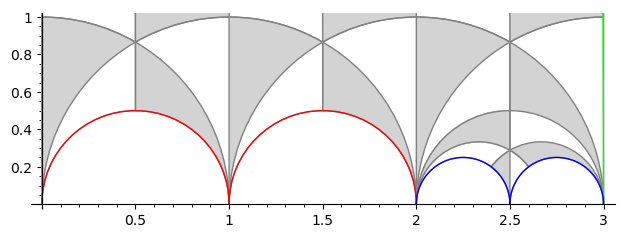# Group given by congruence relationHi,

Beginner in Sage (I love it!) , I want to ask you this maybe naive question:

I have a group $G$ defined by $\lbrace M \in SL_2(Z) \ | \ M = I + kB \ mod(N) \rbrace$, where $I$ is the identity, $N$ a given positive integer, $B$ is a given fixed matrix verifying $B^2 = 0$ and $k$ (not fixed) any integer in { 0,1,2,....,N-1 }. I want to explore conjugacy classes/subgroups of this group $G$ for some specific $B$ and $N$. Is this subgroup finitely generated for some $N$? subgroups of finite index? etc... Do I have a way to do that with SageMath?

Remark that

It is subgroup of a finitely generated group butv that do not imply that it is finitely generated

$B^2 = 0$ gives that $I + kB = \Lambda^k \ with \ \Lambda = I + B$.

An obvious subgroup is $H = \lbrace \Lambda^k, \ k \in \mathbb Z \rbrace$

Any advise or web pointer would be appreciated

edit retag close merge delete

Hi,

Thanks for your interest in my question . Here is the argument

Essentially because $B^2 = 0$.

Just to be clear, saying that $A=B \ mod(N)$ means that $A - B = N \cdot X$ where $X$ is an integral matrix

1) $I \in G$, take k=0

2) Take $A = I + mB + N\cdot X, B = I + nB + N\cdot Y\in G$ , $X,Y$ being any integer coefficients matrices. Write the product and you will see that (because $B^2=0$) we have $A \cdot B = I + (m+n)\cdot B \ mod(N)$

3) For the inverse, you see easily that if $A = 1 + k \cdot B \mod(N)$ then $A^{-1} = 1 - k \cdot B \ mod(N)$

1

So k is not fixed, you should make it clearer in your question.

With this definition, it is the preimage of a subgroup under the reduction mod N: SL(2,Z) -> SL(2,Z/NZ). Hence it is finite index in SL(2,Z) and in particular finitely generated. It is also congruence as it contains Gamma(N).

Yes sorry for that, you are right, fixed. Great thanks for this point of view, gives me a step further.
Yes , in fact $G \cap \Gamma_0 = \Gamma(N)$

Sort by » oldest newest most voted

Here is a function constructing the group

def my_congruence_group(B, N):
M = MatrixSpace(Zmod(N), 2)
B = M(B)
assert (B**2).is_zero()
G = SL(2, Zmod(N))
H = G.subgroup([1 + k*B for k in range(N)])
C = libgap.RightCosets(G, H)

l = libgap.Permutation(G([1,1,0,1]), C, libgap.OnRight)
r = libgap.Permutation(G([1,0,1,1]), C, libgap.OnRight)
s2 = libgap.Permutation(G([0,-1,1,0]), C, libgap.OnRight)
s3 = libgap.Permutation(G([0,1,-1,1]), C, libgap.OnRight)

return ArithmeticSubgroup_Permutation(L=l.sage(), R=r.sage(), S2=s2.sage(), S3=s3.sage())


You can just copy paste the code and use it as

sage: N = 6
sage: B = [2,1,2,4]
sage: G = my_congruence_group(B, N)
sage: G.gens()   # some generators for the group
(
[1 3]  [ 3 -2]  [ 11 -25]
[0 1], [ 2 -1], [  4  -9]
)
sage: print(G.genus())  # the genus of the quotient
0
sage: print(G.nu2(), G.nu3(), G.ncusps())  # elliptic points and cusps on the quotient
0 0 4
sage: F = G.farey_symbol()
sage: F.fundamental_domain()   # plot a fundamental domainYou should have a look atSageMath documentation on subgroups of SL(2,Z) documentation to learn how to use G. in particular the section "Arithmetic subgroups defined by permutations of cosets" which is how I build your group and "Farey Symbol for arithmetic subgroups of PSL2(Z)" which teach you how constructing the fundamental domain works

EDIT: Though I suspect that all your groups are just conjugate to $\Gamma_1(N)$ or $\Gamma_0(N)$ (or at least close enough). The only matrix $B$ with $B^2= 0$ are conjugate to $\begin{pmatrix}0&b\\0&0\end{pmatrix}$.

more

Wonderful, thank you so much for your time and your great answer. You taught me a lot in this last two hours. I will study that with great pleasure.

Hi, Sorry to disturb you again but I have tried various ways and I get always the same error when I run the above code:

Here is an extract of the fullstack just in case :

## Starts with:

GAPError Traceback (most recent call last) .........continue..... ---> 23 G = my_congruence_group(B, N) 24 G.gens() # some generators for the group 25 .........continue..... ends with

GAPError: Syntax error: ] expected in stream:1 [1 1] ^

Thanks for any clue

I guess you are using a too old version of SageMath (I do not remember when this change happend). You could replace G([1,1,0,1]) by [[1,1],[0,1]] (and the same with the other matrices).

Thanks. I have the 8.9 version which is recent. I have tried here: https://sagecell.sagemath.org/ and I got the same error....strange. Don't worries I don't want to waste your time I will do my investigatio and tell you if I find something...Maybe a Python 3 compatibility issue?

Got it. Jesus here is how it works for me...don't ask me why...

def my_congruence_group(B, N): M = MatrixSpace(Zmod(N), 2) print(M)

assert (B**2).is_zero()

B = M(B)
G = SL(2, Zmod(N))
H = G.subgroup([1 + k*B for k in range(N)])
C = libgap.RightCosets(G, H)

M1 = M([[1,1],[0,1]])
M2 = M([[1,0],[1,1]])
M3 = M([[0,-1],[1,0]])
M4 = M([[0,1],[-1,1]])

l = libgap.Permutation( M1, C, libgap.OnRight)
r = libgap.Permutation( M2, C, libgap.OnRight)
s2 = libgap.Permutation( M3, C, libgap.OnRight)
s3 = libgap.Permutation( M4, C, libgap.OnRight)

return ArithmeticSubgroup_Permutation(L=l.sage(), R=r.sage(), S2=s2.sage(), S3=s3.sage())